# The Operator Postulate

With every physical observable there is associated a mathematical operator which is used in conjunction with the wavefunction. Suppose the wavefunction associated with a definite quantized value (eigenvalue) of the observable is denoted by Ψn (an eigenfunction) and the operator is denoted by Q. The action of the operator is given byThe mathematical operator Q extracts the observable value qn by operating upon the wavefunction which represents that particular state of the system. This process has implications about the nature of measurement in a quantum mechanical system. Any wavefunction for the system can be represented as a linear combination of the eigenfunctions Ψn ( see basis set postulate), so the operator Q can be used to extract a linear combination of eigenvalues multiplied by coefficients related to the probability of their being observed (see expectation value postulate).

 Discussion of operators
Index

Schrodinger equation concepts

Postulates of quantum mechanics

 HyperPhysics***** Quantum Physics R Nave
Go Back

# Hermitian Property Postulate

The quantum mechanical operator Q associated with a measurable propertu q must be Hermitian. Mathematically this property is defined by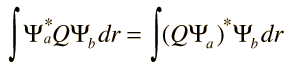where Ψa and Ψb are arbitrary normalizable functions and the integration is over all of space. Physically, the Hermitian property is necessary in order for the measured values (eigenvalues) to be constrained to real numbers.

Index

Schrodinger equation concepts

Postulates of quantum mechanics

 HyperPhysics***** Quantum Physics R Nave
Go Back

# Basis Set Postulate

The set of functions Ψj which are eigenfunctions of the eigenvalue equation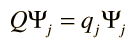form a complete set of linearly independent functions. They can be said to form a basis set in terms of which any wavefunction representing the system can be expressed: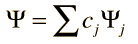This implies that any wavefunction Ψ representing a physical system can be expressed as a linear combination of the eigenfunctions of any physical observable of the system.

Index

Schrodinger equation concepts

Postulates of quantum mechanics

 HyperPhysics***** Quantum Physics R Nave
Go Back

# Expectation Value Postulate

For a physical system described by a wavefunction Ψ , the expectation value of any physical observable q can be expressed in terms of the corresponding operator Q as follows: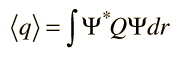It is presumed here that the wavefunction is normalized and that the integration is over all of space. This postulate follows along the lines of the operator postulate and the basis set postulate. The function can be represented as a linear combination of eigenfunctions of Q, and the results of the operation gives the physical values times a probability coefficient. Since the wavefunction is normalized, the integral gives a weighted average of the possible observable values.

 Discussion of expectation values
Index

Schrodinger equation concepts

Postulates of quantum mechanics

 HyperPhysics***** Quantum Physics R Nave
Go Back

# Time Evolution Postulate

If Ψ is the wavefunction for a physical system at an initial time and the system is free of external interactions, then the evolution in time of the wavefunction is given by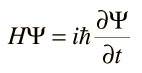where H is the Hamiltonian operator formed from the classical Hamiltonian by substituting for the classical observables their corresponding quantum mechanical operators. For a mechanical system, the classical Hamiltonian would be just the kinetic energy plus the potential energy, i.e., the expression for energy. The role of the Hamiltonian in both space and time is contained in the Schrodinger equation.

Index

Schrodinger equation concepts

Postulates of quantum mechanics

 HyperPhysics***** Quantum Physics R Nave
Go Back